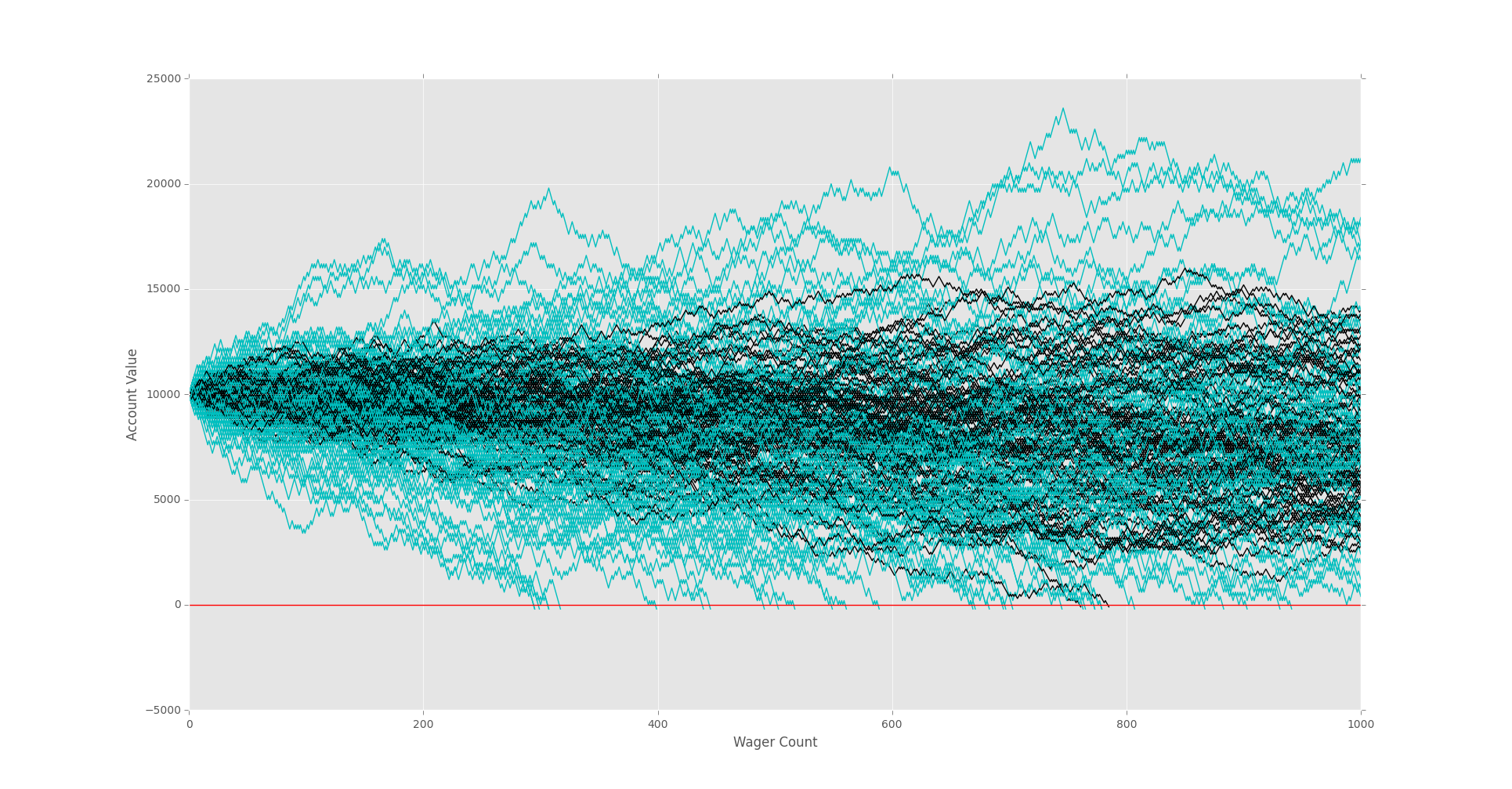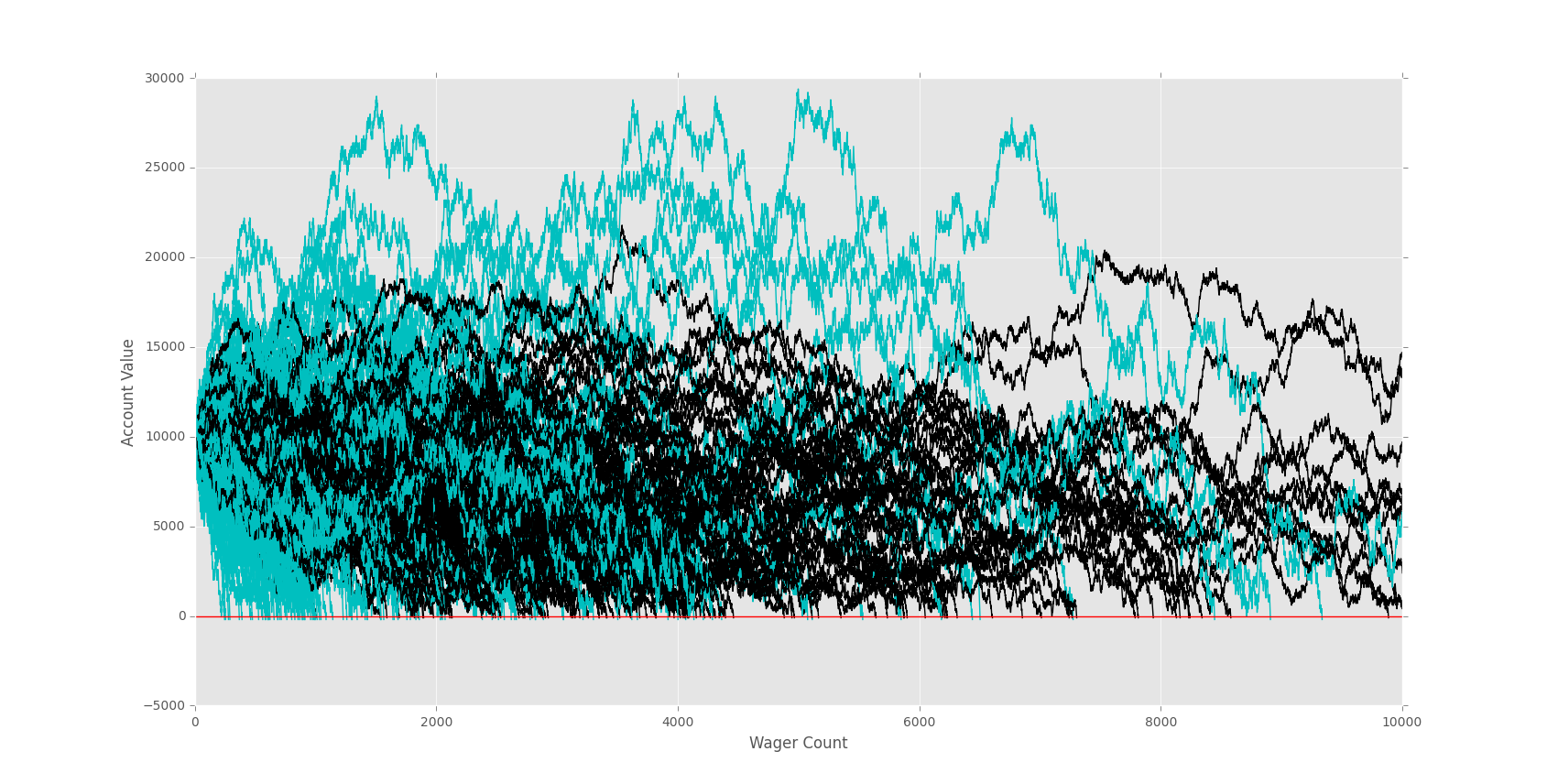## Graphing Monte Carlo

Alright, so we've got our two bettors, we've seen survival rate, but there is more to it than survival rate. Of the survivors, who is the winner, also, is there a point at which a bettor should stop as their odds significantly decrease after a certain point?

Take our doubler bettor for example. Should this doubler bettor actually reach 10x his initial investment, how wise is it that he continues? We are certain that, on a long enough timeline, this doubler will lose everything, so at what point might we be content at our lottery winnings?

Many questions, but first, let's just compare our bettors!

Since we will be comparing bettors, and eventually maybe have a handful, it would be wise to just set the starting funds, wager size, and wager count ahead of time globally.

```import random
import matplotlib
import matplotlib.pyplot as plt
import time

sampleSize = 100

startingFunds = 10000
wagerSize = 100
wagerCount = 1000

def rollDice():
roll = random.randint(1,100)

if roll == 100:
return False
elif roll <= 50:
return False
elif 100 > roll >= 50:
return True

def doubler_bettor(funds,initial_wager,wager_count):

value = funds
wager = initial_wager
wX = []
vY = []
currentWager = 1
previousWager = 'win'
previousWagerAmount = initial_wager

while currentWager <= wager_count:
if previousWager == 'win':
if rollDice():
value += wager
wX.append(currentWager)
vY.append(value)
else:
value -= wager
previousWager = 'loss'
previousWagerAmount = wager
wX.append(currentWager)
vY.append(value)
if value < 0:
currentWager += 10000000000000000
elif previousWager == 'loss':
if rollDice():
wager = previousWagerAmount * 2
value += wager
wager = initial_wager
previousWager = 'win'
wX.append(currentWager)
vY.append(value)
else:
wager = previousWagerAmount * 2
value -= wager
previousWager = 'loss'
previousWagerAmount = wager
wX.append(currentWager)
vY.append(value)
if value < 0:
currentWager += 10000000000000000

currentWager += 1
# this guy goes cyan #
plt.plot(wX,vY,'c')

#####                                           color#
def simple_bettor(funds,initial_wager,wager_count,color):
####

value = funds
wager = initial_wager
wX = []
vY = []
currentWager = 1
while currentWager <= wager_count:
if rollDice():
value += wager
wX.append(currentWager)
vY.append(value)
else:
value -= wager
wX.append(currentWager)
vY.append(value)

if value < 0:
currentWager += 10000000000000000
currentWager += 1

# this guy goes green #
plt.plot(wX,vY,color)

x = 0

while x < sampleSize:
simple_bettor(startingFunds,wagerSize,wagerCount,'k')
simple_bettor(startingFunds,wagerSize*2,wagerCount,'c')
#doubler_bettor(startingFunds,wagerSize,wagerCount)
x+=1

plt.axhline(0, color = 'r')
plt.ylabel('Account Value')
plt.xlabel('Wager Count')
plt.show()
```

Initially, with 1,000 wagers, it looks fairly comparable.Let's look at 10,000 wagers next:On a longer term, our doubler better is clearly dying out. Is it because it is an inferior strategy? Not really. The doubler bettor is just a doubler, and they are increasing the pace by doubling their bets. Thus, they are speeding up the process. That's really all doubling will do for you.

The next tutorial:• Monte Carlo Introduction

• Monte Carlo dice Function

• Creating a simple Bettor

• Plotting Results with Matpltolib

• Martingale Strategy

• Bettor Statistics

• More comparison

• Graphing Monte Carlo
• Fixing Debt Issues

• Analyzing Monte Carlo results

• Using Monte Carlo to find Best multiple

• Checking betting results

• D'Alembert Strategy

• 50/50 Odds

• Analysis of D'Alembert

• Comparing Profitability

• Finding best D'Alembert Multiple

• Two dimensional charting monte carlo

• Monte Carlo Simulation and Python

• Labouchere System for Gambling Tested